# RD Sharma Class 10 Solutions Chapter 15 Statistics Ex 15.2

## RD Sharma Class 10 Solutions Chapter 15 Statistics Ex 15.2

These Solutions are part of RD Sharma Class 10 Solutions. Here we have given RD Sharma Class 10 Solutions Chapter 15 Statistics Ex 15.2

Other Exercises

Question 1.
The. number of telephone calls received at an exchange per interval for 250 successive one-minute intervals are given in the following frequency table: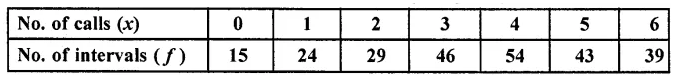Compute the mean number of calls per interval.
Solution:
Let assumed mean (A) = 4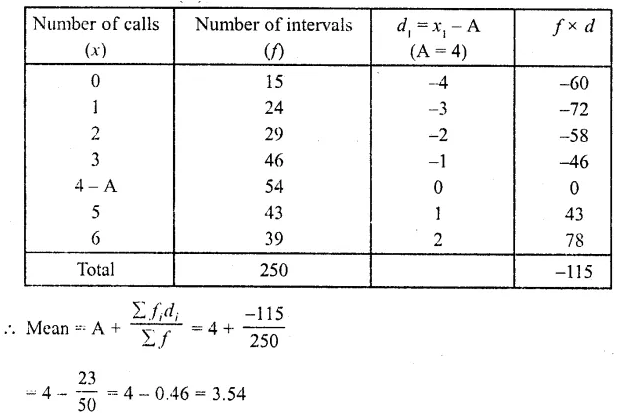Hence mean number of calls per interval = 3.54

Question 2.
Five coins were simultaneously tossed 1000 times, and at each toss the number of heads was observed. The number of tosses during which 0, 1, 2, 3, 4, and 5 heads were obtained are shown in the table below. Find the mean number of heads per toss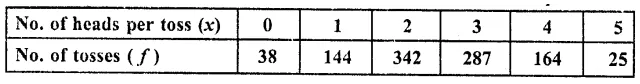Solution:
Let assumed means (A) = 3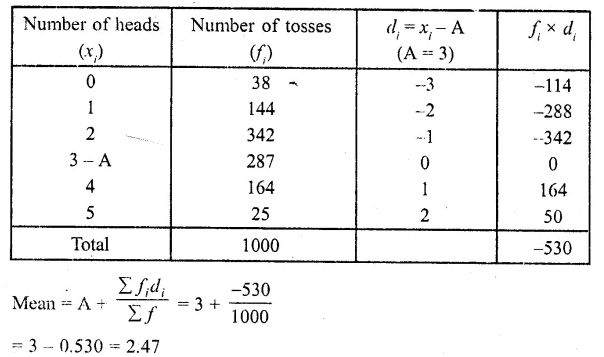Hence mean number of tosses per head = 2.47

Question 3.
The following table gives the number of branches and number of plants in the garden of a school.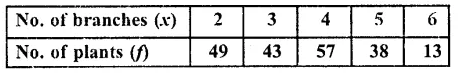Calculate the average number of branches per plant.
Solution:
Let assumed mean (A) = 4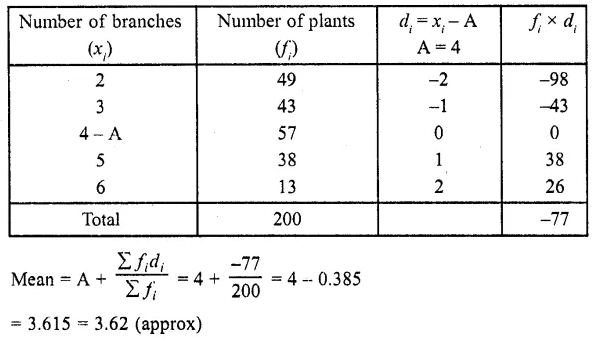∴Mean number of branches per plant = 3.62

Question 4.
The following table gives the number of children of 150 families in a village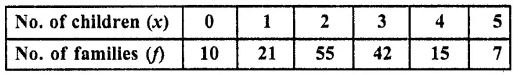Find the average number of children per family.
Solution:
Let assumed mean (A) = 3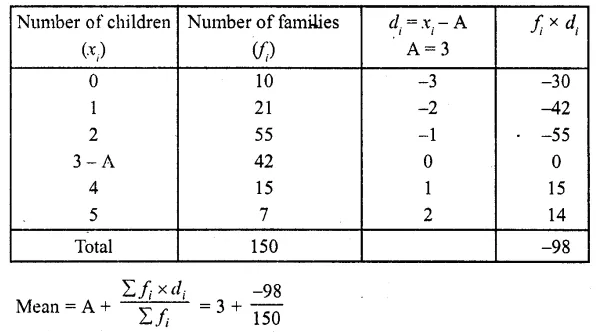= 3 – 0.65 = 2.35
Hence mean number of children per family = 2.35

Question 5.
The marks obtained out of 50, by 102 students in a Physics test are given in the frequency table below: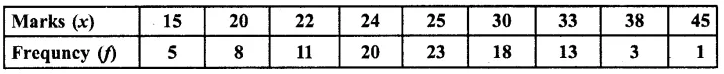Find the average number of marks.
Solution: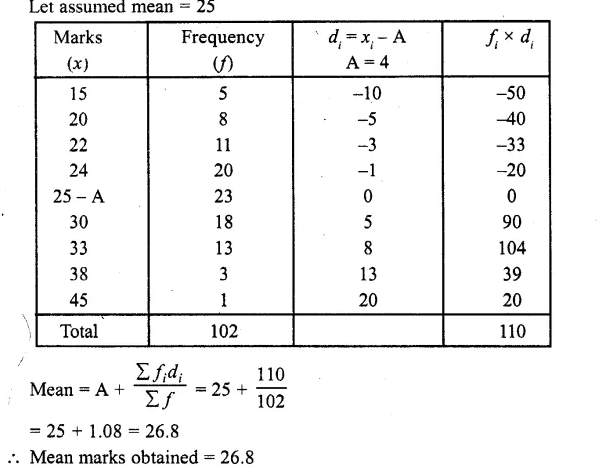Question 6.
The number of students absent in a class were recorded every day for 120 days and the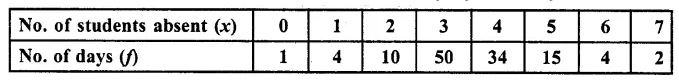Solution:
Let assumed mean = 3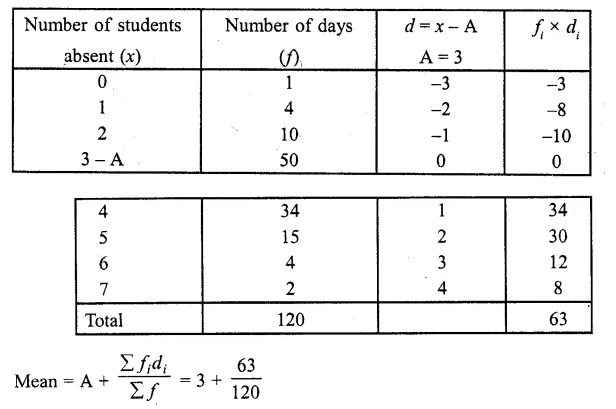= 3 + 0.525 = 3.525 = 3.53 (approx)

Question 7.
In the first proof reading of a book containing 300 pages the following distribution of misprints was obtained: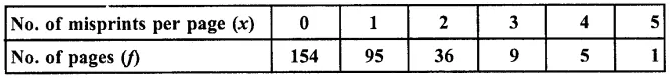Find the average number of misprints per page.
Solution:
Let assumed mean (A) = 2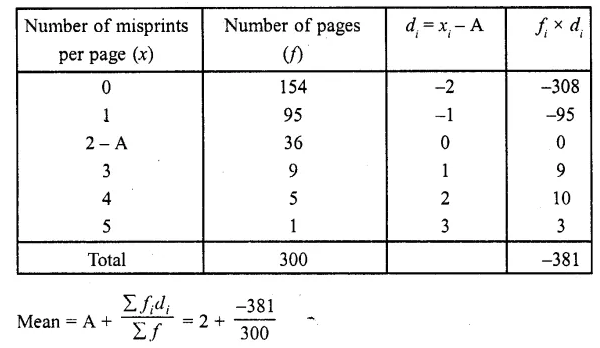= 2 – 127 = 0.73
∴ Average of number of misprints per page = 0.73

Question 8.
The following distribution gives the number of accidents met by 160 workers in a factory during a month.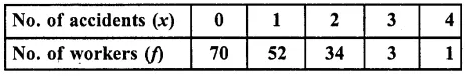Find the average number of accidents per worker.
Solution:
Let assumed mean = 2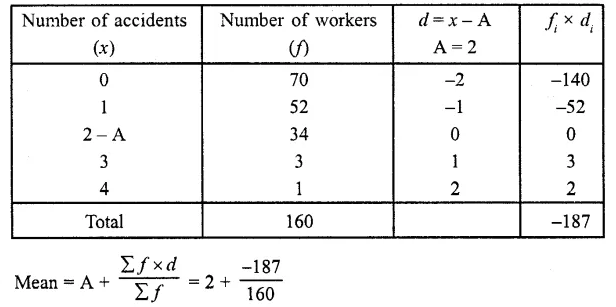= 2 – 1.168 = 2 – 1.17 = 0.83 (approx)
∴ Average number of accidents per worker = 0.83

Question 9.
Find the mean from the following frequency distribution of marks at a test in statistics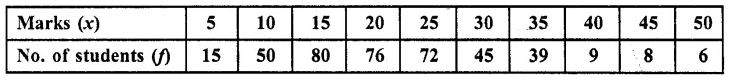Solution:
Let assumed mean = 25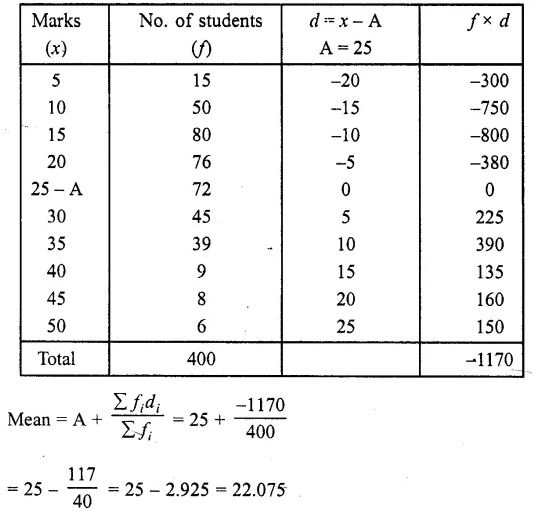∴Average of marked obtained per student = 22.075

Hope given RD Sharma Class 10 Solutions Chapter 15 Statistics Ex 15.2 are helpful to complete your math homework.

If you have any doubts, please comment below. Learn Insta try to provide online math tutoring for you.